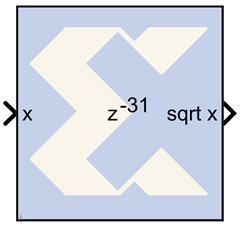# CORDIC SQRT - 2020.2 English

## Vivado Design Suite Reference Guide: Model-Based DSP Design Using System Generator (UG958)

Document ID
UG958
Release Date
2020-11-18
Version
2020.2 English

The Xilinx CORDIC SQRT reference block implements a square root circuit using a fully parallel CORDIC (COordinate Rotation DIgital Computer) algorithm in Hyperbolic Vectoring mode.That is, given input x, it computes the output sqrt (x). The CORDIC processor is implemented using building blocks from the Xilinx blockset.

The square root is calculated indirectly by the CORDIC algorithm by applying the identity listed as follows. sqrt (w) = sqrt { (w + 0.25)2 - (w - 0.25)2 }

The CORDIC square root algorithm is implemented in the following 4 steps:

1. Co-ordinate Rotation: The CORDIC algorithm converges only for positive values of x. If x < zero, the input data is converted to a non-negative number. If x = 0, a zero detect flag is passed to the co-ordinate correction stage. The square root circuit has been designed to converge for all values of x, except for the most negative value.
2. Normalization: The CORDIC algorithm converges only for x between 0.25 (inclusive) and 1. During normalization, the input x is shifted to the left till it has a 1 in the most significant non-signed bit. If the left shift results in an odd number of shift values, a right shift is performed resulting in an even number of left shifts. The shift value is divided by 2 and passed on to the co-ordinate correction stage. The square root is derived using the identity sqrt (w) = sqrt {(w + 0.25)2 - (w - 0.25)2}. Based on this identity the input x gets mapped to, X = x + 0.25 and Y = x - 0.25.
3. Hyperbolic Rotations: For sqrt (X2 - Y2) calculation, the resulting vector is rotated through progressively smaller angles, such that Ygoes to zero.
4. Co-ordinate Correction: If the input was negative and a left shift was applied to x, this step assigns the appropriate sign to the output and multiplies it with 2-shift. If the input was zero, the zero detect flag is used to set the output to 0.

## Block Parameters

The block parameters dialog box can be invoked by double-clicking the icon in your Simulink model.

Parameters specific to this reference block are as follows:

• Number of Processing Elements (integer value starting from 1): specifies the number of iterative stages used for linear rotation.
• Input Data Width: specifies the width of the inputs x. The input x should be signed data type with the same data width as specified.
• Input Binary Point Position: specifies the binary point position for input x. The input x should be signed data type with the specified binary point position.
• Latency for each Processing Element : This parameter sets the pipeline latency after each iterative hyperbolic rotation stage.

The latency of the CORDIC square root block is calculated based on the formula specified below:

Latency = 7 + (data width – binary point)

+ mod { (data width – binary point) , 2 }

+ sum (latency of Processing Elements)

## Reference

• 1) J. E. Volder, The CORDIC Trigonometric Computing Technique, IRE Trans. On Electronic Computers, Vol. EC-8, 1959, pp. 330-334.
• 2) J. S. Walther, A Unified Algorithm for Elementary Functions, Spring Joint Computer Conference (1971) pp. 379-385.
• 3) Yu Hen Hu, CORDIC-Based VLSI Architectures for Digital Signal Processing, IEEE Signal Processing Magazine, pp. 17-34, July 1992.